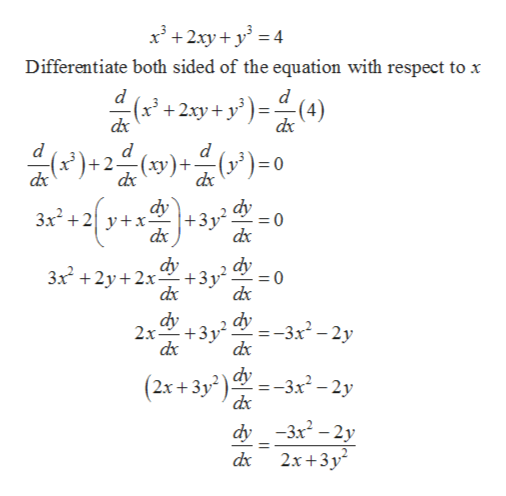# Find an equation of the line tangent to the curve defined by x^3+2xy+y^3=4 at the point (1,1).

Question
22 views

Find an equation of the line tangent to the curve defined by x^3+2xy+y^3=4 at the point (1,1).

check_circle

star
star
star
star
star
1 Rating
Step 1

To find the equation of the tangent line at the point (1, 1) to the curve given below.

Step 2

Formula used: Equation of tangent line to a curve at a point is given by

Step 3

First find the derivative of y with respect to x by implicit...help_outlineImage TranscriptioncloseDifferentiate both sided of the equation with respect to x d d d x+2(y) ) 0 = dx dy dy 3x2 yx +3y2 dx +31,2 dx dx 3x22x 0 dy -3x? - 2у dx 2x +3y =- dx (2x+3y2)3x-2y dx dy -3x-2y 2x+3y dx fullscreen

### Want to see the full answer?

See Solution

#### Want to see this answer and more?

Solutions are written by subject experts who are available 24/7. Questions are typically answered within 1 hour.*

See Solution
*Response times may vary by subject and question.
Tagged in

### Derivative